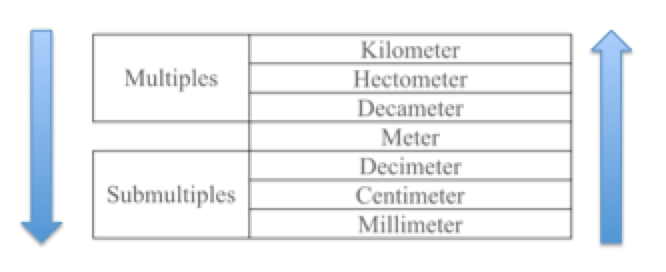Smartick is an advanced online program that teaches kids math and coding in only 15 min. a day

Jan20

# How to Solve Problems with Distance Conversions

In today’s post, we are going to see some problems involving distance conversions, and give some advice for solving them.

#### Problem number 1 with distance conversionsThe pirate, Silver Beard, has arrived on Coral island to search for treasure. On the map, it says that from the shore, he must hopscotch 3.7 hectometers toward the center of the island and then somersault 8.5 more decameters in the same direction. How many meters will he go in total from the shore to the treasure? Write the answer in kilometers.

In order to respond to the question, we must add the two distances, but… can you add hectometers and decameters?

NO, because they are different units!Since they request us to respond in meters, we have to turn both distances into meters. For this, we can adjust this scale to work for us by changing the units. Note that when the order is descending, the units change by multiplying, and when the order is ascending, the units change by dividing.

We see that in order to change from hectometers and decimeters to meters, the arrow goes downward, which means you have to multiply. Therefore:

Since 1 hm = 100 m, Then 3.7 hm = 3.7 x 100 m = 370 m

Since 1 dam= 10 m, Then 8.5 dam = 8.5 x 10 = 85 m

370 m + 85 m = 455 m

We also need to express the solution in kilometers (km). If we look at the table, we see that to go from meters to km the arrow goes upward; therefore, we must divide:

Since 1 km = 1000 m, Then 455 m = 455/1000 km = 0.455 km

Thus, the answer to this distance conversion problem is:

455 meters, which is equal to, 0.455 kilometers.

#### Problem number 2 with distance conversionsGabriela has the most beautiful hair! Before, she used to have the longest hair in the whole class: her hair measured 6 decameters. But yesterday, she cut 25 centimeters, so now the girl in the class with the longest hair is María. How many centimeters long is Gabriela’s hair now? Write the answer in millimeters.

In order to know what the length of her hair is, we must subtract the lengths, but first, they must be converted into the same unit. The unit we need is centimeters, so we have to change the information to cm. As the scale from dm to cm goes down, we must multiply:

Since 1 dm = 10 cm, Then 6 dm = 6 x 10 cm = 60 cm

Now we subtract:

60 cm – 25 cm = 35 cm

In order to express the answer in mm, we have to multiply:

Since 1 cm = 10 mm, Then 35 cm = 35 x 10 mm = 350 mm

Therefore, the answer to this problem is:

35 centimeters, which equals, 350 millimeters.

#### Problem number 3 with distance conversionsA bear that loves honey wants to take honey from a hive, which is on a branch of a tree, but it is too high. In order to reach it, he climbs on a rock that is 12 decameters high directly below the branch, and with claws outstretched, he grabs it. If the bear, standing up, is 2.3 m tall, how far off the ground was the hive?

As in the first problem, in order to solve, it is necessary to add the two distances. But first, we must convert them. However, in this problem, it doesn’t tell us the units that we should use, so we can write the answer in the unit that is easiest for us. We chose meters:

We have to change the dm into m (since the scale ascends, we have to divide by 10):

Since 1 m = 10 dm, Then 12 dm = 1.2 m

1.2 m + 2.3 m = 3.5 m

Therefore, the answer to this problem is:

3.5 meters

How did this post seem to you? Has it helped you to better understand distance conversions?

Well, continue to follow the blog because in the coming weeks we will see more examples of conversion problems dealing with weight and amount. Don’t forget that you can try Smartick for free to learn much more elementary math!